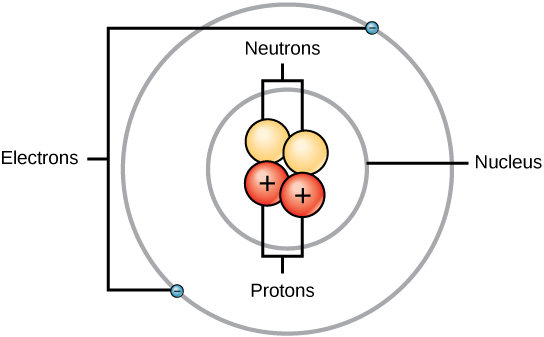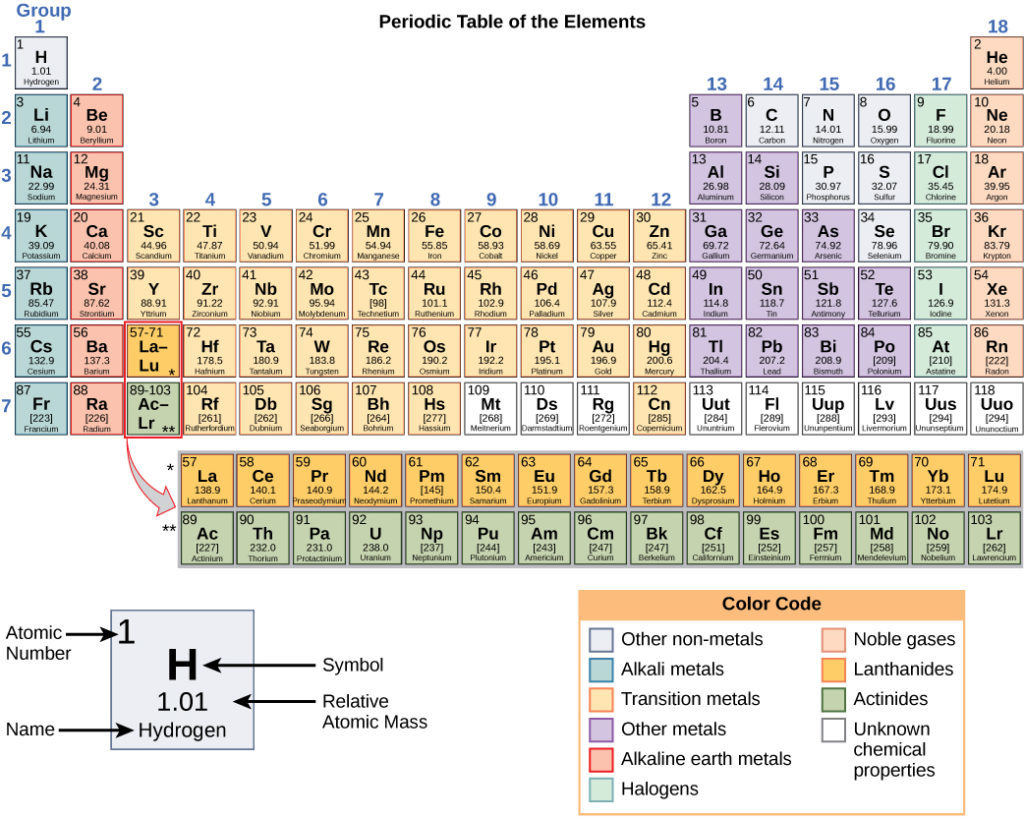## Reading: The Building Blocks of Matter

Why study the chemistry of minerals? Minerals are made of atoms, which have an impact on the behavior and characteristics of the mineral. Thus, to understand, explain, and predict the behavior of minerals, and rocks—which are made of minerals—we must understand some basic facts about atoms and how they behave. This requires a basic understanding of some chemistry. We will begin by constructing atoms in our thinking in terms of the three sub-atomic particles of which atoms are made.

## AtomsFigure 1. Elements, such as helium, depicted here, are made up of atoms. Atoms are made up of protons and neutrons located within the nucleus, with electrons in orbitals surrounding the nucleus.

Atoms consist of protons, neutrons, and electrons. Protons have a positive (+) electrical charge. Electrons have a negative (−) charge that is exactly equal and opposite to the electrical charge of a proton. Neutrons are electrically neutral.

Most of the mass of an atom is packed into its tiny nucleus. An atomic nucleus is made of protons and neutrons, which have approximately the same mass (about 1.67 × 10−24 grams). Electrons, on the other hand, are arranged in specific orbitals around the nucleus of an atom; they are also much smaller in mass than protons and neutrons, weighing only 9.11 × 10−28 grams, or about 1/1800 the weight of protons and neutrons.

Even though the mass of an electron is a tiny mass compared to the mass of a proton or a neutron, the electrons occupy most of the volume of an atom (see Figure 1).

## Ions

A neutral atom has the same number of electrons as it does protons. An atom that has lost or gained any electrons is no longer an electrically neutral atom. That type of atom, which is not electrically neutral and has an electrical charge associated with it, is called an ion. Atoms that have gained electrons are negatively (−) charged ions, or anions. Atoms that have lost electrons are positively (+) charged ions, or cations.

It is also possible to have ions that are actually small groups of atoms bonded together. These are known as polyatomic ions. One example of a polyatomic ion is the carbonate ion, (CO3)2−, which has two extra electrons, giving it the net electrical charge of 2−.

### Chemical Formulas

We’ve just seen that a carbonate ion can also be called (CO3)2−. But what exactly does this mean?

First let’s look at the letters: CO. Atoms have chemical symbols; each element has been assigned one or two letters to represent it. Thus, C stands in for carbon, and O stands in for oxygen (all of these chemical symbols can be seen in the periodic table in figure 2 below).

As we read above, the 2− means that the ion has two extra electrons. But what about the 3? This means there are three oxygen atoms in the ion. The number of atoms in a particular formula is always notated in subscript. Charge is always written in superscript at the end of the formula (a superscript at the beginning of the formula means something else—we’ll get to this when we discuss isotopes below). The parentheses around CO3 indicate that the charge belongs to the whole polyatomic unit rather than just the O3.

Thus the carbonate ion is one carbon atom (C), three oxygen atoms (O3), and two extra electrons (2−), which charge the whole polyatomic ion.

## The Periodic Table

Naturally occurring atoms found in the earth range from hydrogen, with just one proton in its nucleus, to uranium, with 92 protons in its nucleus. These are the naturally occurring chemical elements, which includes such commonly known elements as carbon, oxygen, iron, and so on. The periodic table lists all the chemical elements in a way that tells us how many protons each of them has, how its electrons are arranged, and what the general chemical behavior of each element is.Figure 2. Follow this link to a large version of the Periodic Table. The link will open in a new window so you can easily refer to it as you read through this page.

As shown in Figure 2, the periodic table consists of eighteen groups and seven periods. Two additional rows of elements, known as the lanthanides and actinides, are placed beneath the main table. These elements are placed separately to make the table more compact. All the elements in a group have a similar chemical behavior. This is because all the elements in a group have a similar arrangement of electrons in their atoms, and it is the electron arrangement that determines the chemical behavior of an element.

For each element, the name, atomic symbol, atomic number, and atomic mass are provided. The atomic number is a whole number that represents the number of protons: each chemical element is distinguished by the number of protons in its nucleus. For example, every atom of the element oxygen has eight protons in its nucleus. That is why the atomic number of oxygen is 8. If an atom has greater or fewer than eight protons in its nucleus, it is not oxygen, it is some other chemical element. In the periodic table, the atomic number of each element is listed above the chemical symbol of the element.

The atomic mass, which is the average mass of different isotopes, is estimated to two decimal places. For example, hydrogen has the atomic symbol H, the atomic number 1, and an atomic mass of 1.01. The atomic mass is always larger that the atomic number. For most small elements, the atomic mass is approximately double the atomic number, as the number of protons and neutrons is about equal.

The elements are divided into three categories: metals, nonmetals and metalloids. These form a diagonal line from period two, group thirteen to period seven, group sixteen. All elements to the left of the metalloids are metals, and all elements to the right are nonmetals.

The periodic table was created to help chemists better understand elements and how they function. It is a map to elemental behavior.

## Isotopes

Every atom of a specific element must have the same number of protons in its nucleus. This number is its atomic number. However, there is a range of possible numbers of neutrons it can have in its nucleus. The fact that atoms of a chemical element may have different numbers of neutrons results in each chemical element having several isotopes. Isotopes are atoms of a given chemical element that have different numbers of neutrons in their nuclei.

For example, while all atoms of the element oxygen have eight protons in their nuclei, those oxygen atoms may have eight, nine, or ten neutrons. The different numbers of neutrons in the nucleus distinguishes the three isotopes of oxygen. Oxygen-16 is the isotope of oxygen with 8 neutrons in its nucleus. The number 16 is called the atomic mass number. The atomic mass number is the total number of protons and neutrons in the nucleus of an isotope. From this definition, and knowing that all oxygen atoms have 8 protons in the nucleus, you can deduce that oxygen-17 is the oxygen isotope with 9 neutrons and oxygen-18 is the oxygen isotope with 10 neutrons. Abbreviated into symbols, the three isotopes of oxygen are written as 16O, 17O and 18O.

Isotopes are not very important for understanding minerals, but are important in understanding how to apply chemistry and nuclear physics to geology, such as how to use measurements of radioactive isotopes to measure the ages of rocks and minerals and how to use oxygen isotopes from layers of glacial ice to determine what the temperature of the earth was during an ice age.

## Contribute!

Did you have an idea for improving this content? We’d love your input.